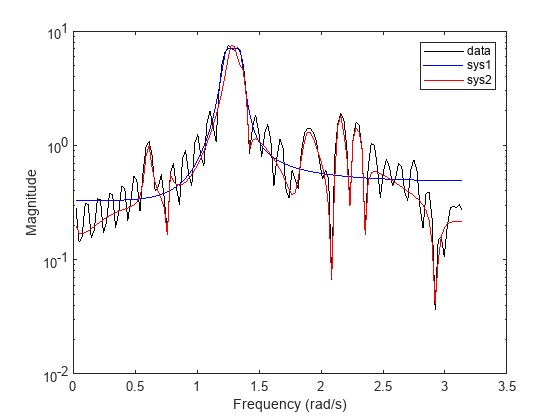# spectrumest

Estimate transfer function model for power spectrum data

## Syntax

``sys = spectrumest(data)``
``sys = spectrumest(data,w)``
``sys = spectrumest(data,w,ts)``
``sys = spectrumest(data,w,ts,np)``
``sys = spectrumest(data,w,ts,np,nz)``
``sys = spectrumest(data,w,ts,Feedthrough=ft)``
``sys = spectrumest(data,w,ts,np,nz,Feedthrough=ft)``
``sys = spectrumest(___,options)``

## Description

### Estimate Model

````sys = spectrumest(data)` fits a minimum-phase, discrete-time transfer function model, `sys`, to the power spectrum data in `data`. The `spectrumest` function assumes that `data` is measured uniformly over a frequency range of 0 to π rad/s. `spectrumest` determines the order (number of poles) of `sys` automatically.```
````sys = spectrumest(data,w)` specifies the frequency vector `w` of the data.```
````sys = spectrumest(data,w,ts)` specifies the sample time `ts` of the data.To estimate discrete-time transfer functions, set ```ts > 0```.To estimate continuous-time transfer functions, set ```ts = 0```.```

example

````sys = spectrumest(data,w,ts,np)` fits a transfer function with `np` poles to the data. `spectrumest` also fits `np` zeros to the data.```
````sys = spectrumest(data,w,ts,np,nz)` fits a transfer function with `np` poles and `nz` zeros to the data.```

### Enable Feedthrough

````sys = spectrumest(data,w,ts,Feedthrough=ft)` specifies whether the identified transfer function `sys` has feedthrough. To enable feedthrough, `sys` must be discrete time (`ts > 0`).```
````sys = spectrumest(data,w,ts,np,nz,Feedthrough=ft)` specifies whether the transfer function with `np` poles and `nz` zeros has feedthrough.```

example

````sys = spectrumest(___,options)` specifies estimation configuration options, such as the numerical search method to be used for estimation. Specify `opt` after all other arguments shown in previous syntaxes.```

## Examples

collapse all

Fit transfer functions models of varying orders to power spectrum data, and compare the resulting spectral models.

Load the benchmark Marple data. Extract the power spectrum from the data, along with the corresponding frequency points and sample time.

```load marple fsys = etfe(marple); ps = squeeze(fsys.SpectrumData); w = fsys.Frequency; Ts = fsys.Ts;```

Fit a fourth-order spectral model to the data.

`sys1 = spectrumest(ps,w,Ts,4);`

Fit a second, more detailed spectral model to the data. Use a `spectrumestOptions` object to apply an inverse weighting filter to the estimated model. Do not specify a number of poles.

```opt = spectrumestOptions(WeightingFilter='inv'); sys2 = spectrumest(ps,w,Ts,opt);```

`spectrumest` generates a plot of model orders (number of poles) to use to estimate the model. The optimal model order identified by `spectrumest` is selected.

1. Under Chosen Order, optionally select a different model order.

2. Click Apply.

Plot the data and the frequency responses of the two spectral models on a semilog plot. The higher-order model produces a more accurate fit to the power spectrum data.

```semilogy(w,sqrt(ps),'k', ... w,squeeze(abs(freqresp(sys1,w))),'b', ... w,squeeze(abs(freqresp(sys2,w))),'r') xlabel('Frequency (rad/s)') ylabel('Magnitude') legend('data','sys1','sys2')```## Input Arguments

collapse all

Power spectrum data, specified as a nonnegative real vector.

Frequency points corresponding to `data`, specified as a numeric vector in units of rad/`TimeUnit`, where `TimeUnit` is the system time unit that was used to compute `data`.

`spectrumest` assumes the Nyquist frequency to be equal to `w(end)`.

Sample time of `data`, in seconds, specified as a nonnegative real scalar.

• If `data` is a continuous-time spectrum, specify `ts = 0`. The output transfer function `sys` has the form

H(s) = num(s) / den(s),

where num and den are the numerator and denominator polynomial coefficients in descending powers of s.

• If `data` is a discrete-time spectrum, specify ```ts > 0``` so that π/`ts` represents the Nyquist frequency. The output transfer function has the form

H(z-1) = num(z-1)/den(z-1),

where num and den are the numerator and denominator polynomial coefficients in descending powers of z-1.

The default value of `ts` depends on whether you specify the spectrum frequencies `w`:

• If you specify `w`, then `ts` = π / `w(end)`.

• If you do not specify `w`, then `ts` = 1.

Number of poles to fit to the transfer function `sys` to determine its model order, specified as one of these values:

• `"best"` — Pick the number of poles required to set an optimal order automatically. The number of poles is in the range `1:length(H)/2`.

• Nonnegative integer — Apply a fixed order to `sys` by fitting the specified number of poles to it.

• Vector of nonnegative integers — Generate a bar plot to pick the optimal order based on the significant singular values of a Loewner matrix generated for the data.

If you specify `np` without `nz`, then `spectrumest` fits `np` zeros to `sys`.

Example: `[1:10]`

Number of zeros to fit to the transfer function `sys`, specified as a nonnegative integer.

• If `data` is a discrete-time spectrum (`ts` > 0), then `nz` represents the number of zeros of the numerator polynomial expressed in the z–1 variable. For example, P(z–1) = 1 + 2z–1 + 3z–2 has 2 zeros.

• If `np` is a scalar value and `nz` is not specified, then `nz` = `np`.

• If `np` is set to `"best"` or a range of values, then the `nz` argument is ignored and instead determined as follows:

• If `ts` = 0 and `ft` is false, then `nz` = `np` – 1.

• In all other cases, `nz` = `np`.

Option to enable feedthrough in transfer function `sys`, specified as logical `1` (`true`) or logical `0` (`false`).

This argument applies only for discrete-time systems (`ts` > 0). For continuous-time systems (`ts` = 0), the presence of feedthrough is implied by `np` = `nz`.

Estimation options, specified as a `spectrumestOptions` option set. Options that you specify include:

• Display of estimation progress

• Weighting prefilter

• Numerical search method to be used in estimation

## Output Arguments

collapse all

Identified spectral model transfer function, returned as an `idtf` object.

## Version History

Introduced in R2022b